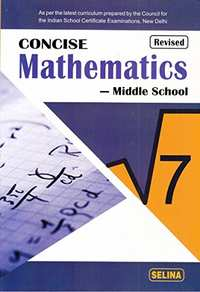# Selina solutions for Concise Mathematics Class 7 ICSE chapter 10 - Simple Interest [Latest edition]

#### Chapters## Chapter 10: Simple Interest

Exercise
Exercise

### Selina solutions for Concise Mathematics Class 7 ICSE Chapter 10 Simple Interest Exercise

Exercise | Q 1.1

Find the S.I. and amount on Rs. 150 for 4 years at 5% per year.

Exercise | Q 1.2

Find the S.I. and amount on Rs. 350 for 3 1/2 years at 8% p.a.

Exercise | Q 1.3

Find the S.I. and amount on Rs. 620 for 4 months at 8 p. per rupee per month.

Exercise | Q 1.4

Find the S.I. and amount on: Rs. 3,380 for 30 months at 4 1/2% p.a.

Exercise | Q 1.5

Find the S.I. and amount on: 600 from July 12 to Dec. 5 at 10% p.a.

Exercise | Q 1.6

Find the S.I. and amount on: Rs. 850 from 10th March to 3rd August at 2 1/2 % p.a.

Exercise | Q 1.7

Find the S.I. and amount on: Rs. 225 for 3 years 9 months at 16% p.a.

Exercise | Q 2

On what sum of money does the S.I. for 10 years at 5% become Rs. 1,600?

Exercise | Q 3

Find the time in which Rs. 2,000 will amount to Rs. 2,330 at 11% p.a.?

Exercise | Q 4

In what time will a sum of money double itself at 8% p.a?

Exercise | Q 5

In how many years will be ₹870 amount to ₹1,044, the rate of interest being 2 % p.a?

Exercise | Q 6

Find the rate percent if the S.I. on ₹275 is 2 years is ₹22.

Exercise | Q 7

Find the sum which will amount to ₹700 in 5 years at 8% rate p.a.

Exercise | Q 8

What is the rate of interest, if ₹3,750 amounts to ₹4,650 in 4 years?

Exercise | Q 9

In 4 years, ₹6,000 amount to ₹8,000. In what time will ₹525 amount to ₹700 at the same rate?

Exercise | Q 10

The interest on a sum of money at the end of 2 years is of the sum. What is the rate percent?

Exercise | Q 11

What sum of money lent out at 5% for 3 years will produce the same interest as Rs. 900 lent out at 4% for 5 years?

Exercise | Q 12

A sum of Rs. 1,780 become Rs. 2,136 in 4 years, Find:
(i) the rate of interest.
(ii) the sum that will become Rs. 810 in 7 years at the same rate of interest?

Exercise | Q 13

A sum amounts to Rs. 2,652 in 6 years at 5% p.a. simple interest. Find:
(i) the sum
(ii) the time in which the same sum will double itself at the same rate of interest.

Exercise | Q 14

P and Q invest Rs. 36,000 and Rs. 25,000 respectively at the same rate of interest per year. If at the end of 4 years, P gets Rs. 3,080 more interest than Q; find the rate of interest.

Exercise | Q 15

A sum of money is lent for 5 years at R% simple interest per annum. If the interest earned be one-fourth of the money lent, find the value of R.

Exercise | Q 16

The simple interest earned on a certain sum in 5 years is 30% of the sum. Find the rate of interest.

## Chapter 10: Simple Interest

Exercise## Selina solutions for Concise Mathematics Class 7 ICSE chapter 10 - Simple Interest

Selina solutions for Concise Mathematics Class 7 ICSE chapter 10 (Simple Interest) include all questions with solution and detail explanation. This will clear students doubts about any question and improve application skills while preparing for board exams. The detailed, step-by-step solutions will help you understand the concepts better and clear your confusions, if any. Shaalaa.com has the CISCE Concise Mathematics Class 7 ICSE solutions in a manner that help students grasp basic concepts better and faster.

Further, we at Shaalaa.com provide such solutions so that students can prepare for written exams. Selina textbook solutions can be a core help for self-study and acts as a perfect self-help guidance for students.

Concepts covered in Concise Mathematics Class 7 ICSE chapter 10 Simple Interest are Concept for Application to Simple Interest (Time Period in Complete Years)., Concept of Principal, Interest, Amount, and Simple Interest.

Using Selina Class 7 solutions Simple Interest exercise by students are an easy way to prepare for the exams, as they involve solutions arranged chapter-wise also page wise. The questions involved in Selina Solutions are important questions that can be asked in the final exam. Maximum students of CISCE Class 7 prefer Selina Textbook Solutions to score more in exam.

Get the free view of chapter 10 Simple Interest Class 7 extra questions for Concise Mathematics Class 7 ICSE and can use Shaalaa.com to keep it handy for your exam preparation